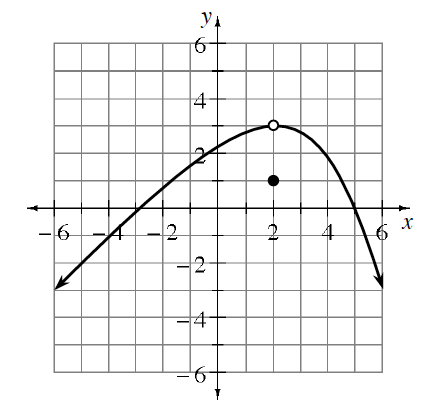### Home > PC3 > Chapter 10 > Lesson 10.1.4 > Problem10-88

10-88.

Use the graph of $y = f(x)$ at right to evaluate each of the following expressions.

1. $\lim\limits_ { x \rightarrow 2 ^ { - } } f ( x )$

This is the limit as x approaches $2$ from the left. Start on the curve at $x = 0$ and move towards $x = 2$. What height is the function approaching?

1. $\lim\limits_ { x \rightarrow 2 ^ { + } } f ( x )$

This is the limit as $x$ approaches $2$ from the right.

1. $\lim\limits_ { x \rightarrow 2 } f ( x )$

This limit exists if your answers to parts (a) and (b) are the same.

1. $f(2)$

This is the value of the function at $x = 2$. A function is not defined when there is an open dot.

1. Is the function continuous at $x = 2$? Use your answers to parts (a) through (d) to justify your conclusion.

A function is continuous at a point if the limit exists and is equal to the function value at that point.